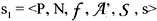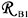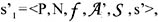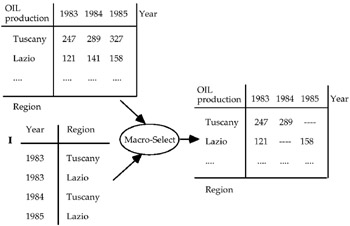# MACRO-SELECT

This is a unary operator, proposed in Fortunato et al. (1986), which selects one or more single values of the measure depending on the relation I given in input. The result is a new MAD defined on the same MAD schema and the same definition domains of the MAD category attributes, with its descriptive space equal to the active domain (see Maier, 1983) of the relation I. No recomputation of the measure instances is required.

Therefore, letbe the considered MAD and let I the relation formed by a subset of tuples of the base relation(which forms the descriptive space of s1). The macro-select is carried out depending on if the relation I is the new MAD, where the only changing is the measure, in which the only summary attribute values, characterized by the tuples of the relation I, appear. The other summary values are substituted by the null value ‘-.’

An example is shown in Figure 14.Figure 14Multidimensional Databases: Problems and Solutions
ISBN: 1591400538
EAN: 2147483647
Year: 2003
Pages: 150